图像处理 幂律变换_数字图像处理中的幂律变换matalb - CSDN
• 图像伽马变化的基本形式为： ... 伽马变换可以很好地拉伸图像的对比度，扩展灰度级。由图可知，当图像的整体灰度偏暗时，选择y 增亮；当图像整体灰度偏亮时，选择y>1，可以使图像变暗，提高图像的对比度，凸显细节。
    图像伽马变化的基本形式为：
s=cr^y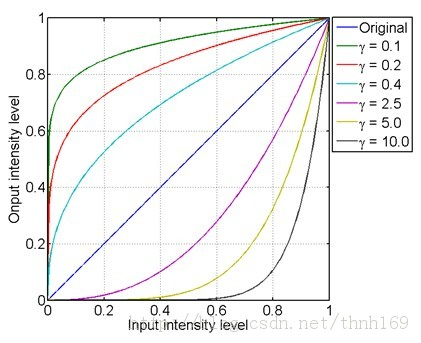伽马变换可以很好地拉伸图像的对比度，扩展灰度级。由图可知，当图像的整体灰度偏暗时，选择y<1,可以使图像
增亮；当图像整体灰度偏亮时，选择y>1，可以使图像变暗，提高图像的对比度，凸显细节。

展开全文• 本系列python版本：python3.5.4 本系列opencv-python版本：opencv-python3.4.2.17 本系列使用的开发环境是jupyter notebook，是一个python的交互式开发环境，测试十分方便，并集成了...上文【数字图像处理系列二...

本系列python版本：python3.5.4
本系列opencv-python版本：opencv-python3.4.2.17
本系列使用的开发环境是jupyter notebook，是一个python的交互式开发环境，测试十分方便，并集成了vim操作，安装教程可参考：windows上jupyter notebook主题背景、字体及扩展插件配置(集成vim环境)

上文【数字图像处理系列二】基本概念：亮度、对比度、饱和度、锐化、分辨率 中我们一起学习了数字图像概念和形成原理，并在实践和对比中一起分析了图像亮度、对比度、饱和度、锐化、分辨率等属性。图像的基础知识介绍完毕，此后将开始着重介绍数字图像处理和分析，本文便从 图像灰度变换增强 开始说起，这在图像预处理中也可以说是很重要的了，杠~~

老规矩，先上图：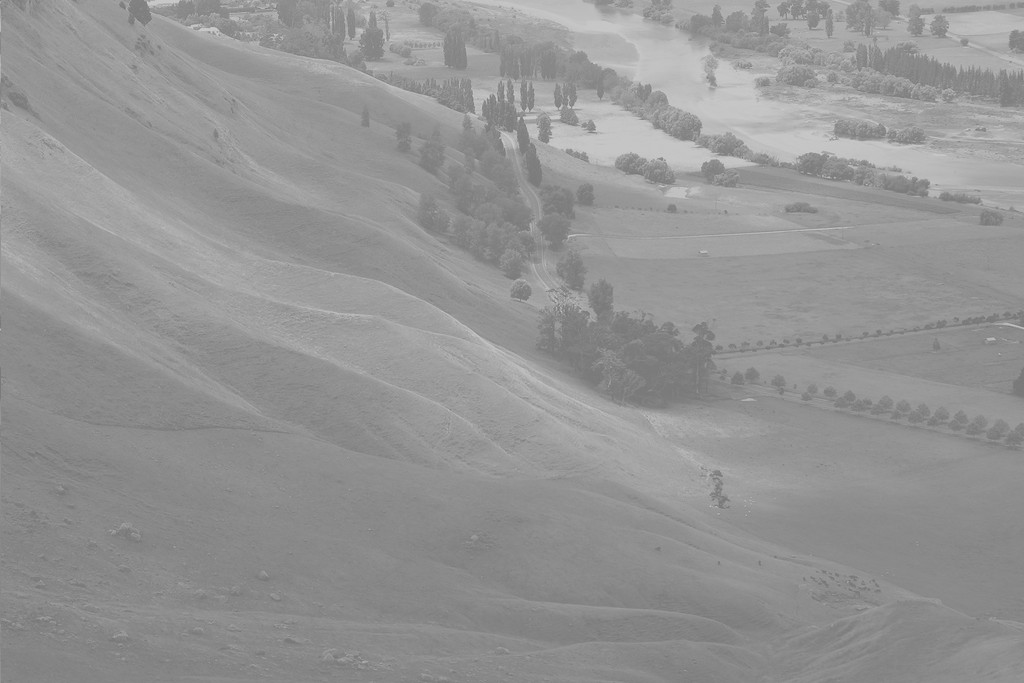经过图像增强后的图片：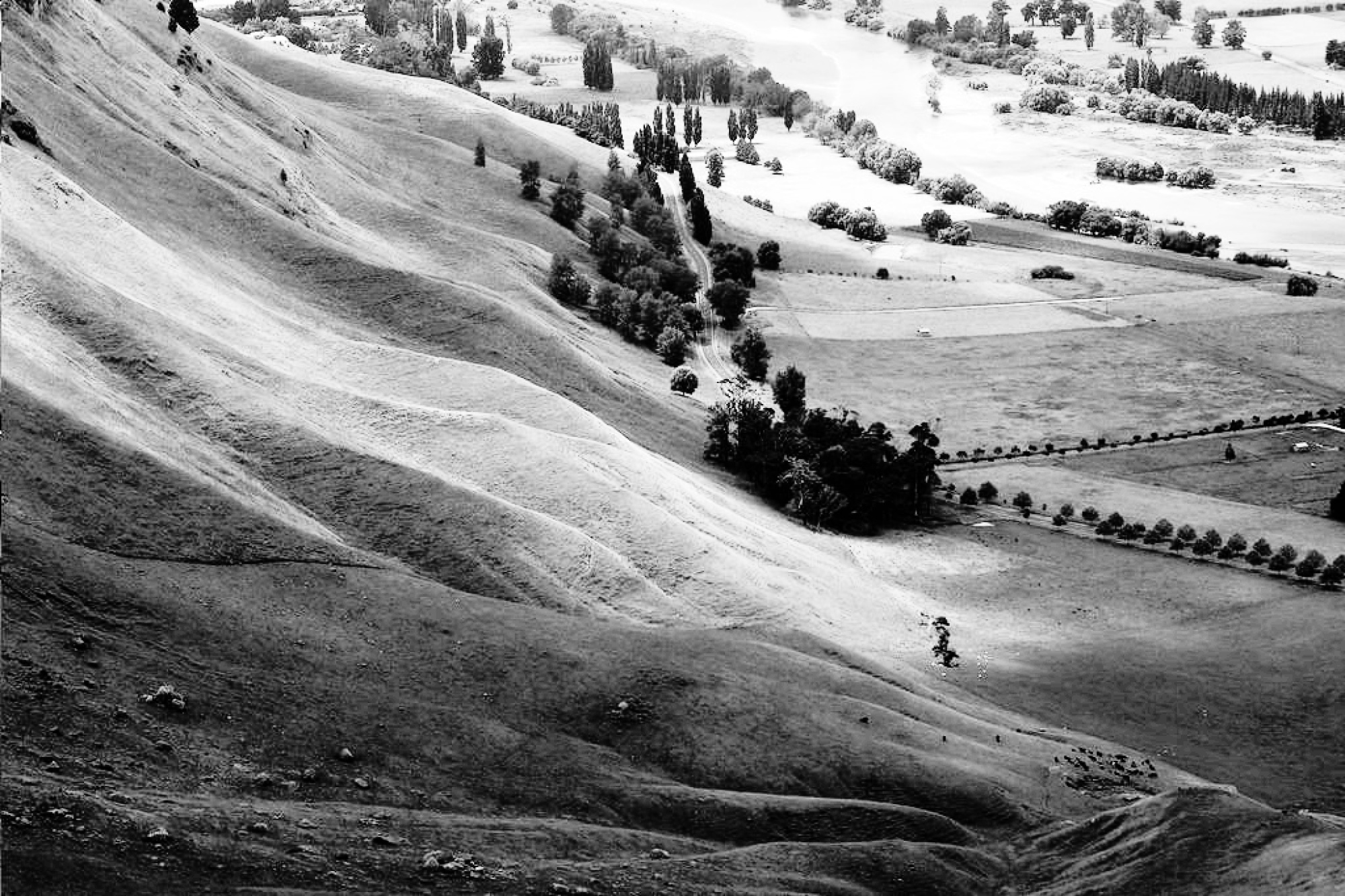一、图像增强知识预热

1、为啥要做图像增强
在图像形成的过程中，存在很多因素影响图像的清晰度，列举几个常见影响图像清晰度因素如下：

光照不够均匀，这会造成图像灰度过于集中
由CCD(摄像头)获得图像时金A/D(数模)转换、线路传送时产生噪声污染，影响图像质量

因此必须在图像处理分析之前对图像的质量进行改善，改善方法分为两类：

图像增强
图像复原

图像增强不考虑图像质量下降的原因，只将图像中感兴趣的特征有选择的突出，而衰减不需要的特征，它的目的主要是提高图像的可懂度； 图像复原技术与增强技术不同，它需要了解图像质量下降的原因，首先要建立"降质模型"，再利用该模型，恢复原始图像。
本文便重点研究一下图像增强技术，图像增强主要是以对比度和阈值处理为目的，且都是在空间域进行的操作。我们假设 f(x，y) 是输入图像，g(x，y) 为经过增强变换处理后的图像，则有：这里的 T 便是在点 (x，y) 的邻域定义的关于 f 的算子，根据邻域的不同，图像增强分为两种：

邻域处理技术
点处理技术，又称 图像灰度变换增强技术，本文重点将讨论

2、图像增强邻域处理技术
空间域处理 g(x，y) = T[f(x， y)] 中点 (x，y) 便是图像的像素坐标，而邻域是中心在 (x，y) 的矩形，其尺寸比图像要小得多，如下图所示：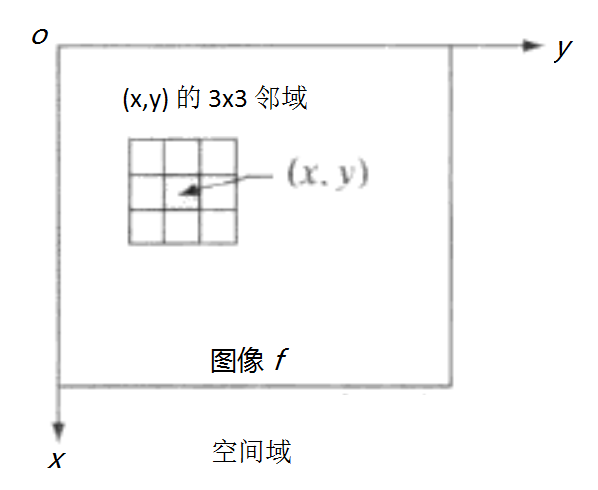图像增强邻域处理技术的核心思想便是： 当对 (x，y) 做变换时，变换算子 T 是对以 (x，y) 为中心的邻域作用然后得出变换结果，如上图，假如该邻域的大小是3*3的正方形，算子 T 定义为 计算该邻域的平均灰度，如 (x，y) = (100，200)，则 g(100，200) 的结果便是计算  f(100，200) 和它的8个邻点的和，然后在除以9 (即是由邻域包围的像素灰度的平均值)，当然这种邻域处理思想在后续的图像滤波处理中是基本应用，如均值滤波、中值滤波和高斯滤波等都是采用邻域处理技术。

3、图像增强点处理技术
点处理技术，既是当邻域的大小为 1*1 时，此时 g 的值仅仅取决于点 (x，y) 处的值，此时 T 称为灰度变换函数，此种图像增强方式就是图像灰度变换增强，定义公式如下：其中 s 是变换后的像素，r 是变换前的像素，此后讨论的线性变换、 分段线性变换、 对数变换、 反对数变换、 幂律(伽马)变换均是基于此公式为基础进行的，因此图像灰度变换便是基于点的增强方式，它将每一个像素的灰度值按照一定的数学变换公式转换为一个新的灰度值，达到增强图像的效果。

二、图像灰度变换增强

说在前面：  线性变换和分段线性变换一般对灰度图像进行处理，也可以直接对RGB图像进行处理；对数变换、 反对数变换、 幂律(伽马)变换则是直接对RGB图像进行相应的图像增强，达到自己想要的结果
如果对RGB图像进行变换之后需要多两部操作：(1) 图像归一化、(2) 图像像素值类型转化为np.uint8，需要用到两个函数，在这里做简单介绍，后续示例程序中将会使用到：

函数cv2.normalize()
#数字图像归一化操作
cv2.normalize(src, dst, alpha, beta, norm_type, dtype, mask) → dst

参数解释：

src： 原图像对象

dst： 经过转化后的图像对象

alpha： 归一化后灰度像素最小值，一般为0

beta： 归一化后灰度像素最大值，一般为255

norm_type： 归一化的类型，可以取以下值
(1) cv2.NORM_MINMAX： 数组的数值被平移或缩放到一个指定的范围，线性归一化，一般较常用
(2) cv2.NORM_INF:此类型的定义没有查到，根据OpenCV 1的对应项，可能是归一化数组的C-范数(绝对值的最大值)
(3) cv2.NORM_L1 :  归一化数组的L1-范数(绝对值的和)
(4) cv2.NORM_L2: 归一化数组的(欧几里德)L2-范数

dtype： 为负数时，输出图像像素值的type与输入相同，一般使用默认

函数cv2.convertScaleAbs()
#该函数较简单，便是将原图像的像素值均转化成类型为np.uint8
dst = cv2.convertScaleAbs(src)


1、线性变换
图像增强线性变换主要可以对图像的对比度和亮度进行调整，线性变换公式如下：参数 a 影响图像的对比度，参数 b 影响图像的亮度，具体分为可分为以下几种情况：

a>1： 增强图像的对比度，图像看起来更加清晰
a<1： 减小了图像的对比度， 图像看起来变暗
a<0 and b=0：图像的亮区域变暗，暗区域变亮
a=1 and b≠0：图像整体的灰度值上移或者下移，也就是图像整体变亮或者变暗，不会改变图像的对比度，b>0时图像变亮，b<0时图像变暗
a=-1 and b=255：图像翻转

上代码：
import cv2
import random
import imutils
import numpy as np

#彩色图像每个像素值是[x,y,z], 灰度图像每个像素值便是一个np.uint8
gray_img = cv2.cvtColor(image, cv2.COLOR_BGR2GRAY)

#图像大小调整
ori_h, ori_w = image.shape[:2]
height, width = gray_img.shape[:2]
image = cv2.resize(image, (int(ori_w/ori_h*400), 400), interpolation=cv2.INTER_CUBIC)
gray_img = cv2.resize(gray_img, (int(width/height*400), 400), interpolation=cv2.INTER_CUBIC)

#a<0 and b=0: 图像的亮区域变暗，暗区域变亮
a, b = -0.5, 0
new_img1 = np.ones((gray_img.shape, gray_img.shape), dtype=np.uint8)
for i in range(new_img1.shape):
for j in range(new_img1.shape):
new_img1[i][j] = gray_img[i][j]*a + b

#a>1: 增强图像的对比度,图像看起来更加清晰
a, b = 1.5, 20
new_img2 = np.ones((gray_img.shape, gray_img.shape), dtype=np.uint8)
for i in range(new_img2.shape):
for j in range(new_img2.shape):
if gray_img[i][j]*a + b > 255:
new_img2[i][j] = 255
else:
new_img2[i][j] = gray_img[i][j]*a + b

#a<1: 减小了图像的对比度, 图像看起来变暗
a, b = 0.5, 0
new_img3 = np.ones((gray_img.shape, gray_img.shape), dtype=np.uint8)
for i in range(new_img3.shape):
for j in range(new_img3.shape):
new_img3[i][j] = gray_img[i][j]*a + b

#a=1且b≠0, 图像整体的灰度值上移或者下移, 也就是图像整体变亮或者变暗, 不会改变图像的对比度
a, b = 1, -50
new_img4 = np.ones((gray_img.shape, gray_img.shape), dtype=np.uint8)
for i in range(new_img4.shape):
for j in range(new_img4.shape):
pix = gray_img[i][j]*a + b
if pix > 255:
new_img4[i][j] = 255
elif pix < 0:
new_img4[i][j] = 0
else:
new_img4[i][j] = pix

#a=-1, b=255, 图像翻转
new_img5 = 255 - gray_img

cv2.imshow('origin', imutils.resize(image, 800))
cv2.imshow('gray', imutils.resize(gray_img, 800))
cv2.imshow('a<0 and b=0', imutils.resize(new_img1, 800))
cv2.imshow('a>1 and b>=0', imutils.resize(new_img2, 800))
cv2.imshow('a<1 and b>=0', imutils.resize(new_img3, 800))
cv2.imshow('a=1 and b><0', imutils.resize(new_img4, 800))
cv2.imshow('a=-1 and b=255', imutils.resize(new_img5, 800))
if cv2.waitKey(0) == 27:
cv2.destroyAllWindows()


代码运行效果，原灰度图像：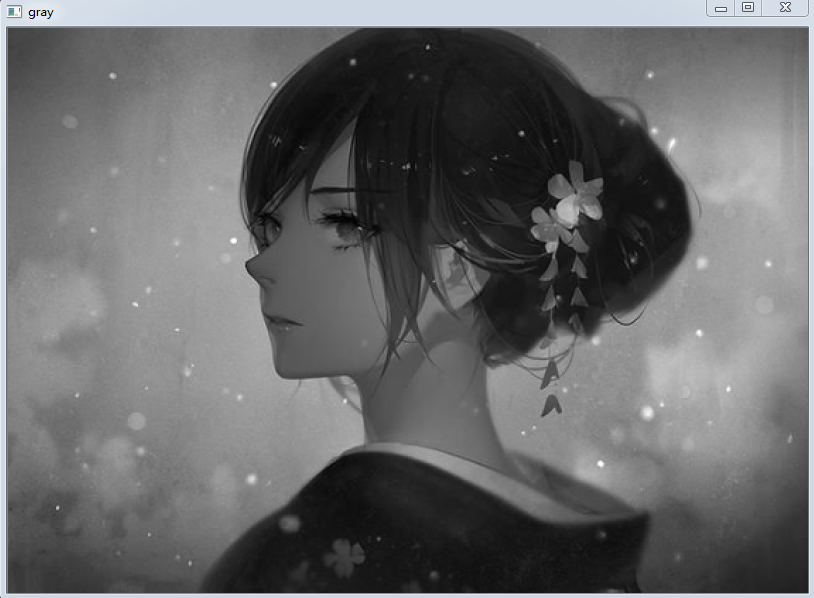通过对图像对比度、亮度增强之后效果如下：注： 明白一点就是图像增强从来没有通用的理论，在不同的场景下会有不同的需求，图像灰度增强最简单的线性变换可以满足对图像对比度和亮度进行随意的调整，得到自己想要的结果

2、分段线性变换
分段线性变换函数来增强图像对比度的方法实际是增强原图各部分的反差，即增强输入图像中感兴趣的灰度区域，相对抑制那些不感兴趣的灰度区域。增分段线性函数的主要优势在于它的形式可任意合成，而其缺点是需要更多的用户输入。分段线性函数通用公式如下：下面我们便通过实例来感受一下分段线性函数对于图像增强的几个方面的应用，并体会上面公式的含义！

2.1   分段线性变换应用之对比度拉伸、阈值处理
低对比度图像一般由光照不足，成像传感器动态范围太小，图像获取过程中镜头光圈设置错误引起，对比度拉伸是扩展图像灰度级动态范围的处理。变换算子 T 如下所示：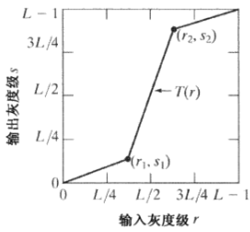根据r1、s1、r2、s2可分为以下两种增强情况：

r1 <= r2、s1 <= s2： 对比度拉伸，增强感兴趣区域
r1 = r2： 阈值处理，产生一个二值图像

(1) 对比度拉伸实例演示
下面令(r1， s1) = (rmin，0)、(r2， s2) = (rmax，L-1)，其中 rmin、rmax 分别代表数字图像中最小灰度级和最大灰度级，此变换函数将灰度级由原来范围线性拉伸到整个范围[0，L-1]， 上示例代码：
import cv2
import imutils
import numpy as np

gray_img = cv2.cvtColor(image, cv2.COLOR_BGR2GRAY)

#在灰度图进行分段线性对比度拉伸
#此种方式变换函数把灰度级由原来的线性拉伸到整个范围[0, 255]
r_min, r_max = 255, 0
for i in range(gray_img.shape):
for j in range(gray_img.shape):
if gray_img[i, j] > r_max:
r_max = gray_img[i, j]
if gray_img[i, j] < r_min:
r_min = gray_img[i, j]
r1, s1 = r_min, 0
r2, s2 = r_max, 255

precewise_img = np.zeros((gray_img.shape, gray_img.shape), dtype=np.uint8)
k1 = s1/r1
k3 = (255-s2)/(255-r2)
k2 = (s2 - s1)/(r2 - r1)
for i in range(gray_img.shape):
for j in range(gray_img.shape):
if r1 <= gray_img[i, j] <= r2:
precewise_img[i, j] = k2*(gray_img[i, j] - r1)
elif gray_img[i, j] < r1:
precewise_img[i, j] = k1*gray_img[i, j]
elif gray_img[i, j] > r2:
precewise_img[i, j] = k3*(gray_img[i, j] - r2)

cv2.imshow('origin image', imutils.resize(image, 480))
cv2.imshow('precewise image', imutils.resize(precewise_img, 480))
if cv2.waitKey(0) == 27:
cv2.destroyAllWindows()


上述代码是在灰度图片上进行的图像增强，我们也可以直接在原图上进行图像增强，显示出来的效果都是相同的，代码如下：
import cv2
import imutils
import numpy as np

#直接在原图上进行分段线性对比度拉伸
#此种方式变换函数把灰度级由原来的线性拉伸到整个范围[0, 255]
r_min, r_max = 255, 0
for i in range(image.shape):
for j in range(image.shape):
for k in range(image.shape):
if image[i, j, k] > r_max:
r_max = image[i, j, k]
if image[i, j, k] < r_min:
r_min = image[i, j, k]
r1, s1 = r_min, 0
r2, s2 = r_max, 255
k1 = s1/r1
k3 = (255-s2)/(255-r2)
k2 = (s2 - s1)/(r2 - r1)

precewise_img = np.zeros((image.shape, image.shape, 3), dtype=np.float32)
for i in range(image.shape):
for j in range(image.shape):
for k in range(image.shape):
if r1 <= image[i, j, k] <= r2:
precewise_img[i, j, k] = k2*(image[i, j, k] - r1)
elif image[i, j, k] < r1:
precewise_img[i, j, k] = k1*gray_img[i, j, k]
elif image[i, j, k] > r2:
precewise_img[i, j, k] = k3*(gray_img[i, j, k] - r2)

#原图中做分段线性变化后需要对图像进行归一化操作，并将数据类型转换到np.uint8
cv2.normalize(precewise_img, precewise_img, 0, 255, cv2.NORM_MINMAX)
precewise_img = cv2.convertScaleAbs(precewise_img)

cv2.imshow('origin image', imutils.resize(image, 480))
cv2.imshow('precewise image', imutils.resize(precewise_img, 480))
if cv2.waitKey(0) == 27:
cv2.destroyAllWindows()


花粉原图和经过对比度拉伸后的图像效果如下：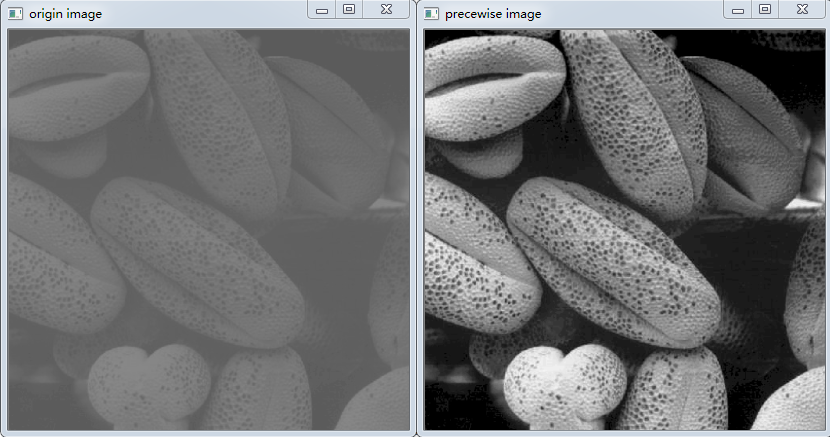(2) 阈值处理实例演示
import cv2
import imutils
import numpy as np

gray_img = cv2.cvtColor(image, cv2.COLOR_BGR2GRAY)

#阈值处理函数：当r1=r2, s1=0, s2=L-1时，此时分段线性函数便是阈值处理函数
plist = []
for i in range(gray_img.shape):
for j in range(gray_img.shape):
plist.append(gray_img[i, j])
r_avg = int(sum(plist)/len(plist))
thresh_img = np.zeros((gray_img.shape, gray_img.shape), dtype=np.uint8)
for i in range(gray_img.shape):
for j in range(gray_img.shape):
if gray_img[i, j] < r_avg:
thresh_img[i, j] = 0
else:
thresh_img[i, j] = 255

cv2.imshow('origin image', imutils.resize(image, 480))
cv2.imshow('thresh image', imutils.resize(thresh_img, 480))
if cv2.waitKey(0) == 27:
cv2.destroyAllWindows()


经过阈值处理后的花粉图像如下所示: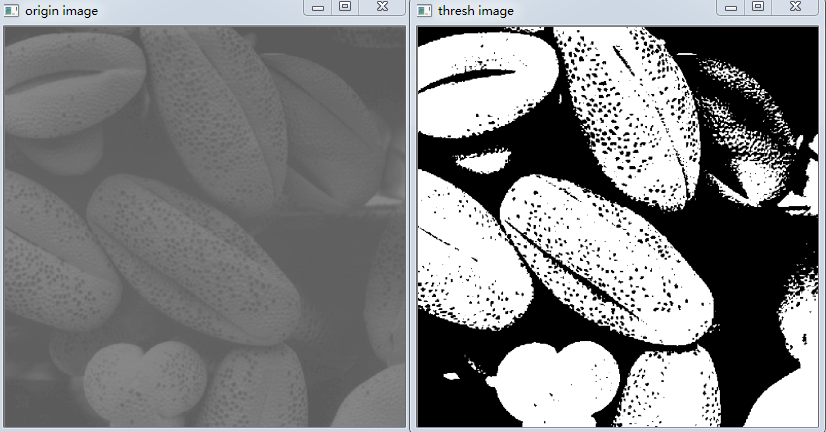2.2   分段线性变换应用之灰度级分层
为了在数字图像中突出我们感兴趣的灰度级区域 [A，B]，在实际情况下可以有两种处理方式，对应下图：

突出灰度范围在 [A，B] 的区域，将其他区域灰度级降低到一个更低的级别
突出灰度范围在 [A，B] 的区域，其他区域保持原灰度级不变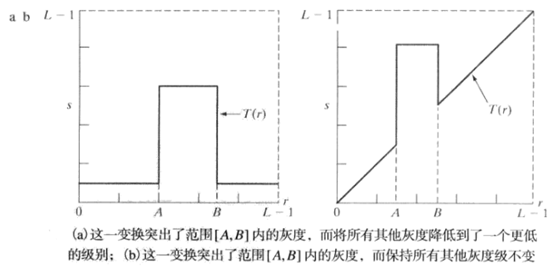上示例代码(下面展示的是：其他区域保持原灰度级不变的情况)
import cv2
import imutils
import numpy as np

#在某一范围(A, B)突出灰度，其他灰度值保持不变
gray_img = cv2.cvtColor(image, cv2.COLOR_BGR2GRAY)

r_left, r_right = 150, 230
r_min, r_max = 0, 255
level_img = np.zeros((gray_img.shape, gray_img.shape), dtype=np.uint8)
for i in range(gray_img.shape):
for j in range(gray_img.shape):
if r_left <= gray_img[i, j] <= r_right:
level_img[i, j] = r_max
else:
level_img[i, j] = gray_img[i, j]

cv2.imshow('origin image', imutils.resize(image, 480))
cv2.imshow('level image', imutils.resize(level_img, 480))
if cv2.waitKey(0) == 27:
cv2.destroyAllWindows()


原图和效果图如下所示：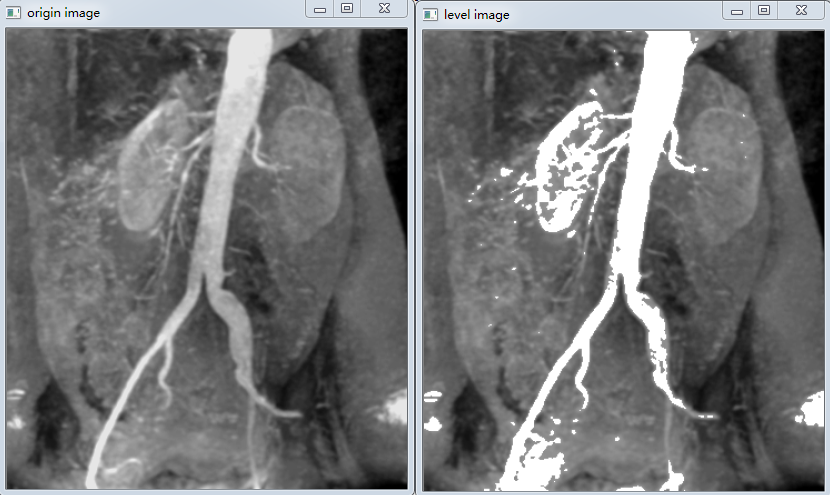3、对数变换
对数变换将图像的低灰度值部分扩展，将其高灰度值部分压缩，以达到强调图像低灰度部分的目的；同时可以很好的压缩像素值变化较大的图像的动态范围，目的是突出我们需要的细节。反对数变换则与对数函数不同的是，强调的是图像的高灰度部分，对数变换公式如下：下面示例将演示对数变换：
import cv2
import imutils
import numpy as np

log_img = np.zeros((image.shape, image.shape, 3), dtype=np.float32)
for i in range(image.shape):
for j in range(image.shape):
log_img[i, j, 0] = math.log(1 + image[i, j, 0])
log_img[i, j, 1] = math.log(1 + image[i, j, 1])
log_img[i, j, 2] = math.log(1 + image[i, j, 2])
cv2.normalize(log_img, log_img, 0, 255, cv2.NORM_MINMAX)
log_img = cv2.convertScaleAbs(log_img)
cv2.imshow('image', imutils.resize(image, 400))
cv2.imshow('log transform', imutils.resize(log_img, 400))
if cv2.waitKey(0) == 27:
cv2.destroyAllWindows()


傅里叶频谱和经过对数变换增强后的频谱图像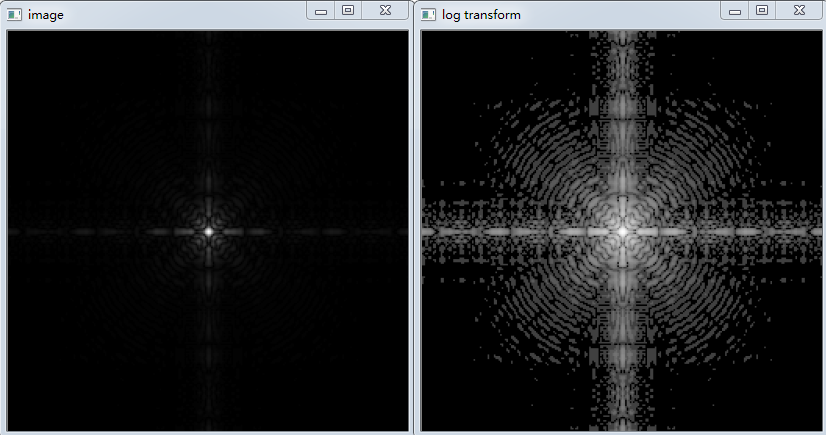4、幂律变换
幂律变换主要用于图像的校正，对漂白的图片或者是过黑的图片进行修正，幂律变换的公式如下：根据 φ 的大小，主要可分为一下两种情况：

φ > 1： 处理漂白的图片，进行灰度级压缩
φ < 1： 处理过黑的图片，对比度增强，使得细节看的更加清楚

4.1  当φ > 1时示例如下：
#幂律变换 φ>1
gamma_img1 = np.zeros((image.shape, image.shape, 3), dtype=np.float32)
for i in range(image.shape):
for j in range(image.shape):
gamma_img1[i, j, 0] = math.pow(image[i, j, 0], 5)
gamma_img1[i, j, 1] = math.pow(image[i, j, 1], 5)
gamma_img1[i, j, 2] = math.pow(image[i, j, 2], 5)
cv2.normalize(gamma_img1, gamma_img1, 0, 255, cv2.NORM_MINMAX)
gamma_img1 = cv2.convertScaleAbs(gamma_img1)
cv2.imshow('image', imutils.resize(image, 400))
cv2.imshow('gamma1 transform', imutils.resize(gamma_img1, 400))
if cv2.waitKey(0) == 27:
cv2.destroyAllWindows()


漂白图片和经过幂律变换后图像对比效果：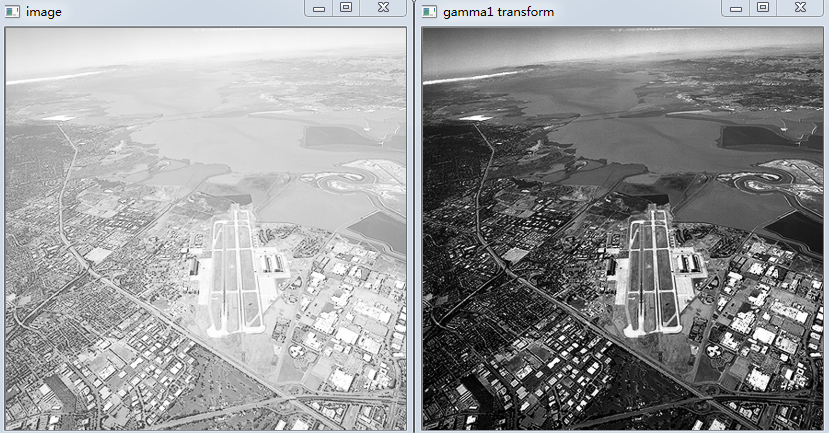4.2  当φ< 1时示例如下：
#幂律变换，φ<1
gamma_img2 = np.zeros((image.shape, image.shape, 3), dtype=np.float32)
for i in range(image.shape):
for j in range(image.shape):
gamma_img2[i, j, 0] = math.pow(image[i, j, 0], 0.4)
gamma_img2[i, j, 1] = math.pow(image[i, j, 1], 0.4)
gamma_img2[i, j, 2] = math.pow(image[i, j, 2], 0.4)
cv2.normalize(gamma_img2, gamma_img2, 0, 255, cv2.NORM_MINMAX)
gamma_img2 = cv2.convertScaleAbs(gamma_img2)
cv2.imshow('image', imutils.resize(image, 400))
cv2.imshow('gamma2 transform', imutils.resize(gamma_img2, 400))
if cv2.waitKey(0) == 27:
cv2.destroyAllWindows()


过黑图片和经过幂律变换后效果展示图：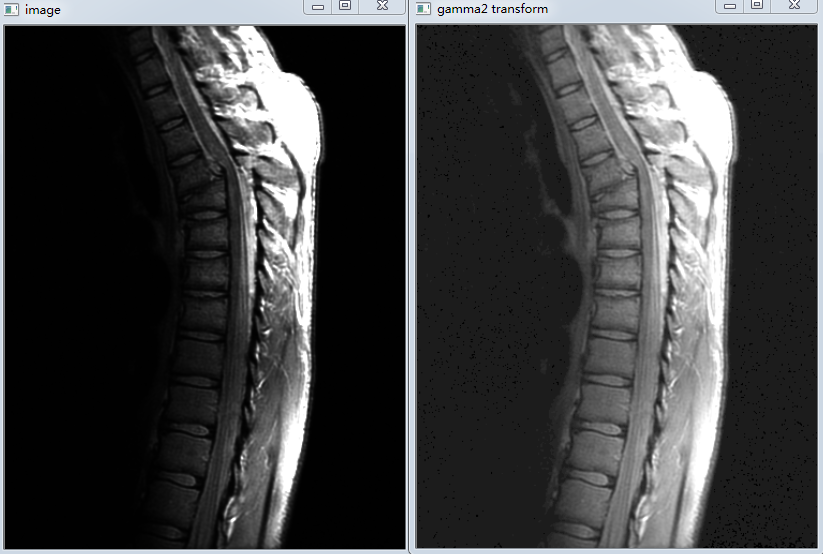三、直方图均衡化

说在前面：  数字图像直方图均衡化目的就是提升图像的对比度，将较亮或者较暗区域的输入像素映射到整个区域的输出像素，是图像增强一种很好的且方便的方式，因此单独列为一节进行讲解

1、认识图像的直方图
图像直方图：反应图像强度分布的总体概念，宽泛的来说直方图给出了图像对比度、亮度和强度分布信息。下面从理论的角度阐述一下图像直方图的公式定义
对于灰度级范围为[0，L-1]的数字图像的直方图是离散函数：其中 rk 是第k级灰度值，nk 便是图像中灰度为 rk 的像素个数。当然如果数字图像长宽维度为MN，则对直方图归一化之后的公式为：下面我们先从直观的角度来感受一下不同图像对应的直方图的区别，这对后续直方图均衡化增强图像理解有很大的帮助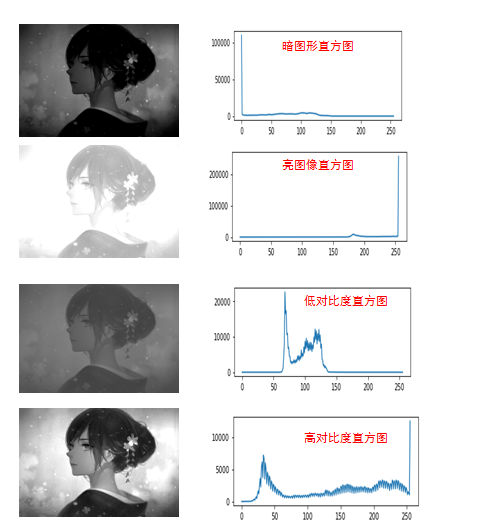从上图中我们注意到： 在暗图像中，直方图的分布都集中在灰度级的低(暗)端； 亮图像直方图的分布集中在灰度级的高端；低对比度图像具有较窄的直方图，且都集中在灰度级的中部；而高对比度的图像直方图的分量覆盖了很宽的灰度范围，且像素分布也相对均匀，此时图片的效果也相对很不错。于是我们可以得出结论：若一幅图像的像素倾向于占据整个可能的灰度级并且分布均匀，则该图像有较高的对比度并且图像展示效果会相对好，于是便引出图像直方图均衡化，对图像会有很强的增强效果

2、图像增强之直方图均衡化
这里不详细介绍直方图均衡化原理，想了解请看博客： 直方图均衡化原理
下面给出直方图均衡化的实现：
import cv2
import imutils
import numpy as np
import matplotlib.pyplot as plt

wiki_gray = cv2.cvtColor(wiki_img, cv2.COLOR_BGR2GRAY)

#对图像进行均衡化处理，增强图像对比度
wiki_equ = cv2.equalizeHist(wiki_gray)

hist = cv2.calcHist([wiki_gray], , None, , [0, 256])
equ_hist = cv2.calcHist([wiki_equ], , None, , [0, 256])
fig = plt.figure()
ax1.plot(hist)
ax2.plot(equ_hist)
plt.show()

cv2.imshow('wiki_origin', imutils.resize(wiki_img, 400))
cv2.imshow('wiki_gray', imutils.resize(wiki_gray, 400))
cv2.imshow('wiki_equ', imutils.resize(wiki_equ, 400))
if cv2.waitKey(0) == 27:
cv2.destroyAllWindows()


直方图均衡化斥候：直方图前后对比图、图像前后对比图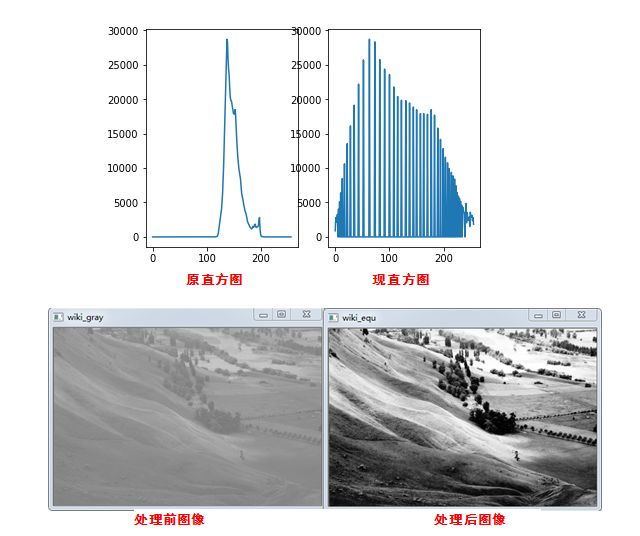四、总结

不总结了，本篇分享到此结束，码字码到眼睛疼，如果诸位看管觉得还可以帮忙点个赞，后续将持续更新数字图像处理和分析等操作，也欢迎关注博主微信公众号展开全文线性变换 分段线性变换 对数变换 幂律变换 直方图均衡化
• 图像幂律变换处理，当γ取值（0,1）可以将图像较暗的灰度值映射到更大的灰度值，同时较亮部分变化较小；γ取值（1，+∞）可以将较亮部分映射到更低的亮度，而更暗的部分变化较小。 处理彩色图像，只需要将RGB...
        幂律(伽马)变换公式：，γ取值范围（0，+∞）

对图像做幂律变换处理，当γ取值（0,1）可以将图像较暗的灰度值映射到更大的灰度值，同时较亮部分变化较小；γ取值（1，+∞）可以将较亮部分映射到更低的亮度，而更暗的部分变化较小。

处理彩色图像，只需要将RGB取出，分别进行幂律变换即可。公式中，c可以取值255，r = a/255，a为像素上RGB分量之一，那么整个公式的取值范围为（0,255），不考虑计算过程的四舍五入的话，幂律变换的操作是可逆的。

实现代码如下：

public void gammaTranverse(BufferedImage image, double param) {
for (int i = 0; i < image.getWidth(); i++) {
for (int j = 0; j < image.getHeight(); j++) {

// 将rgb转换为各个值
int rgb = image.getRGB(i, j);
double R = (rgb >> 16) & 0xff;
double G = (rgb >> 8) & 0xff;
double B = rgb & 0xff;

// 进行伽马变换
R = 255 * Math.pow(R / 255, param);
G = 255 * Math.pow(G / 255, param);
B = 255 * Math.pow(B / 255, param);
// 将值转换为rgb
rgb = ((clamp(255) & 0xff) << 24) | ((clamp((int) R) & 0xff) << 16) | ((clamp((int) G) & 0xff) << 8)
| ((clamp((int) B) & 0xff));
image.setRGB(i, j, rgb);
}
}
}

测试结果：

1、原图。这是一张光线暗到几乎很难直接看到地面物体的航拍影像。通过幂律变换进行处理，增强较暗影像。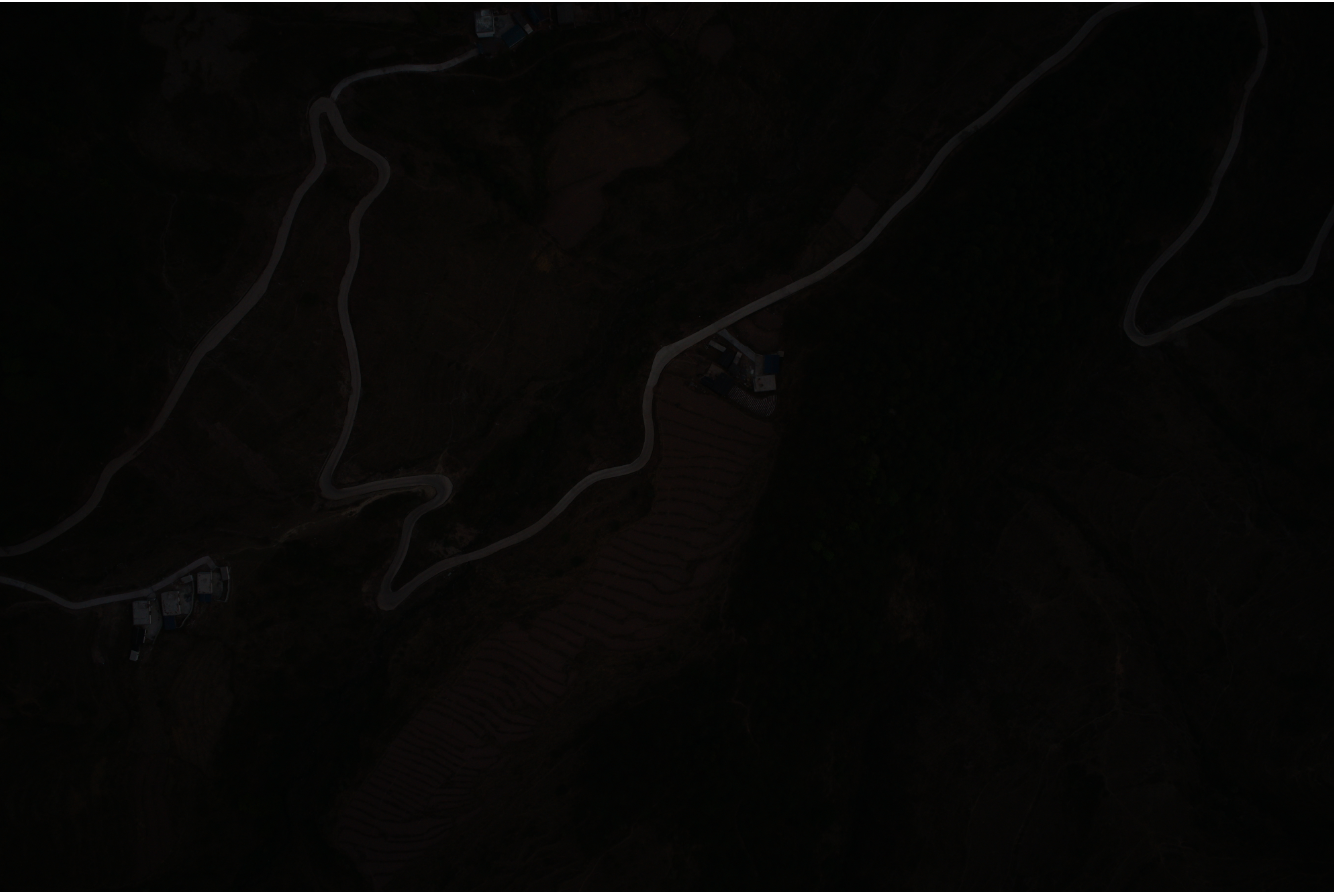2、γ取值为0.4，通过幂律变换处理后效果，可以看到影像增强效果非常明显，影像大部分区域由几乎不可见变得可见。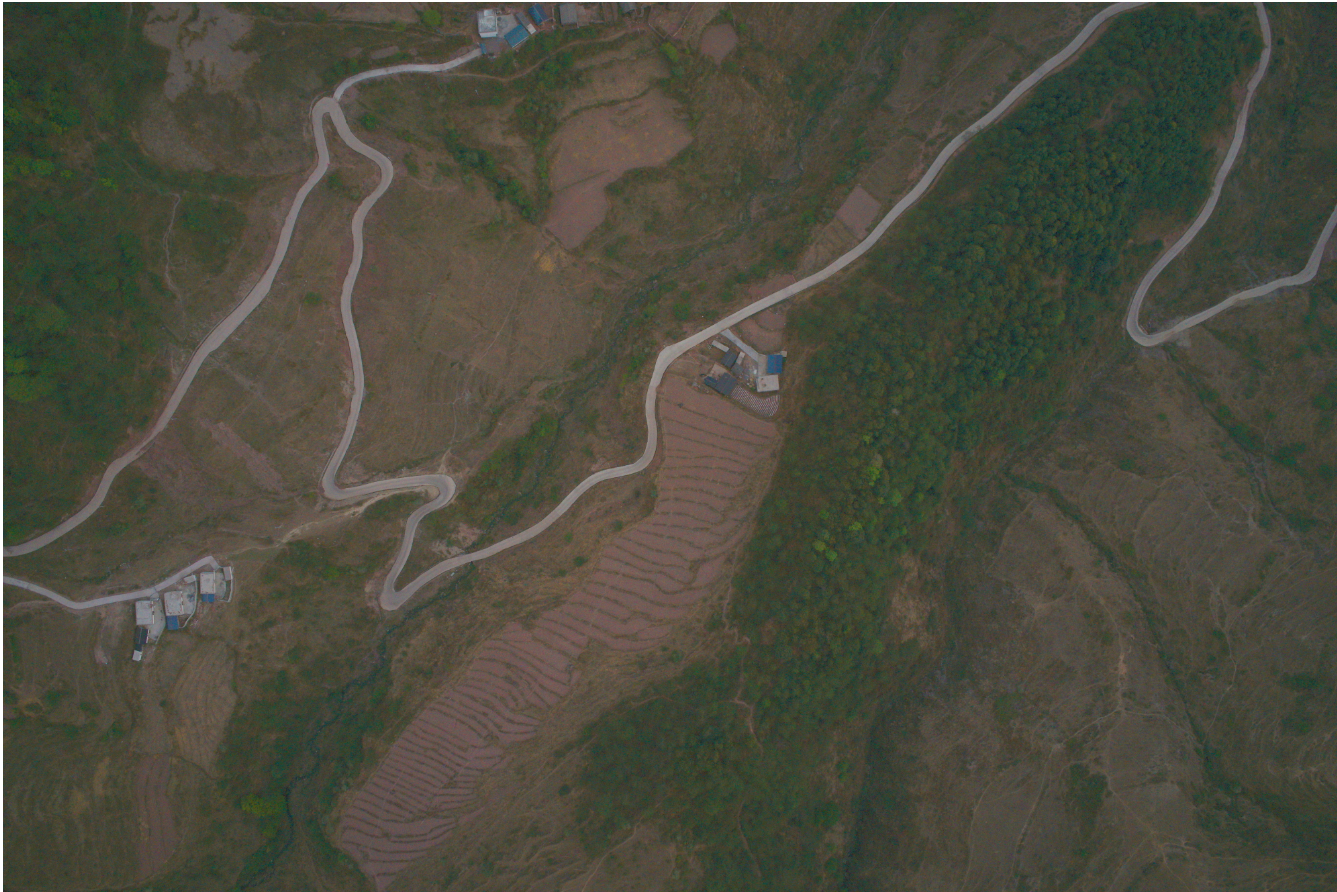3、这是一幅光线比较强，且受到云雾干扰的影像。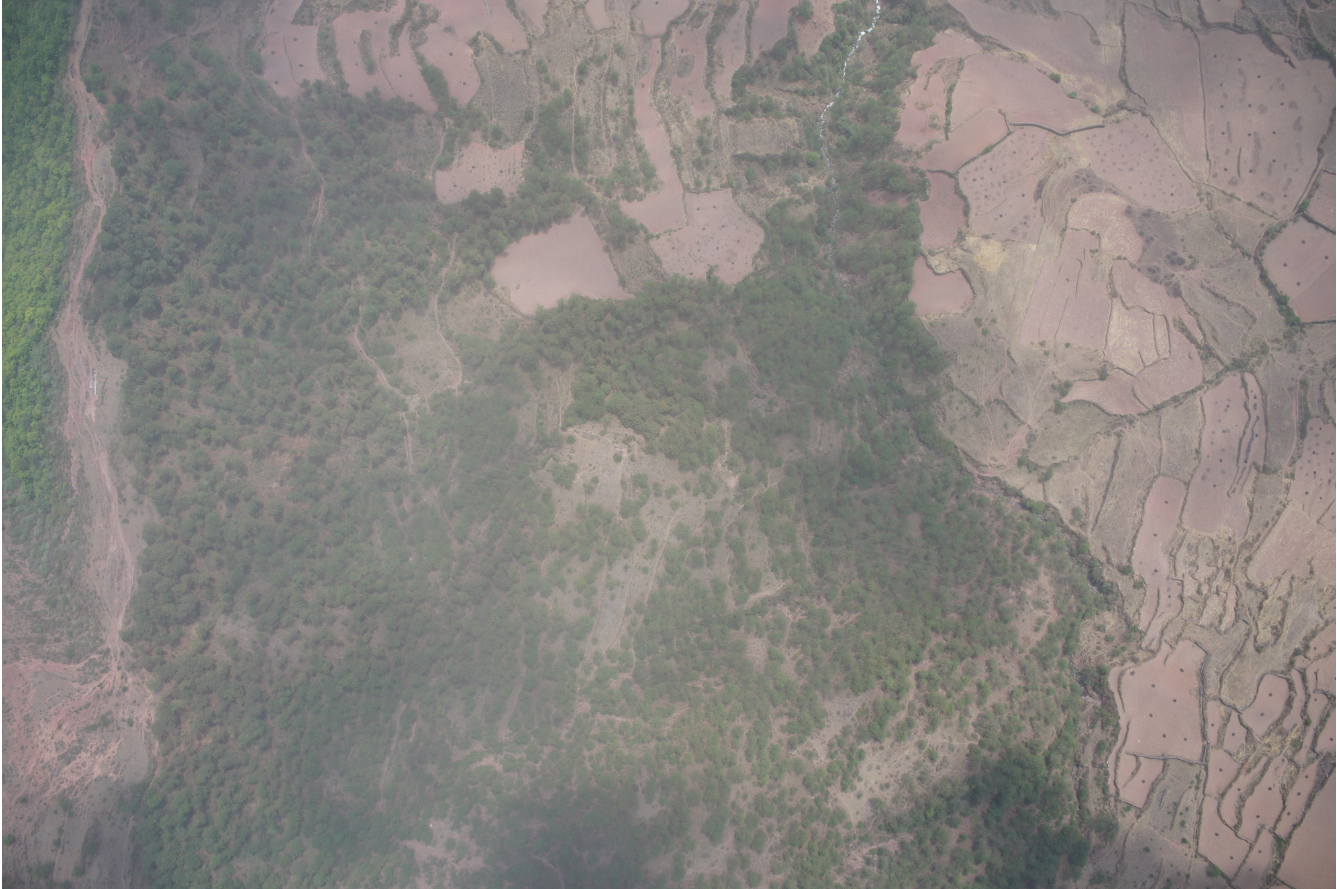4、γ取值为2处理后效果，影像整体变暗，但对比度更明显，受光线和云雾干扰造成的泛白现象减弱，整体视觉效果有所提升。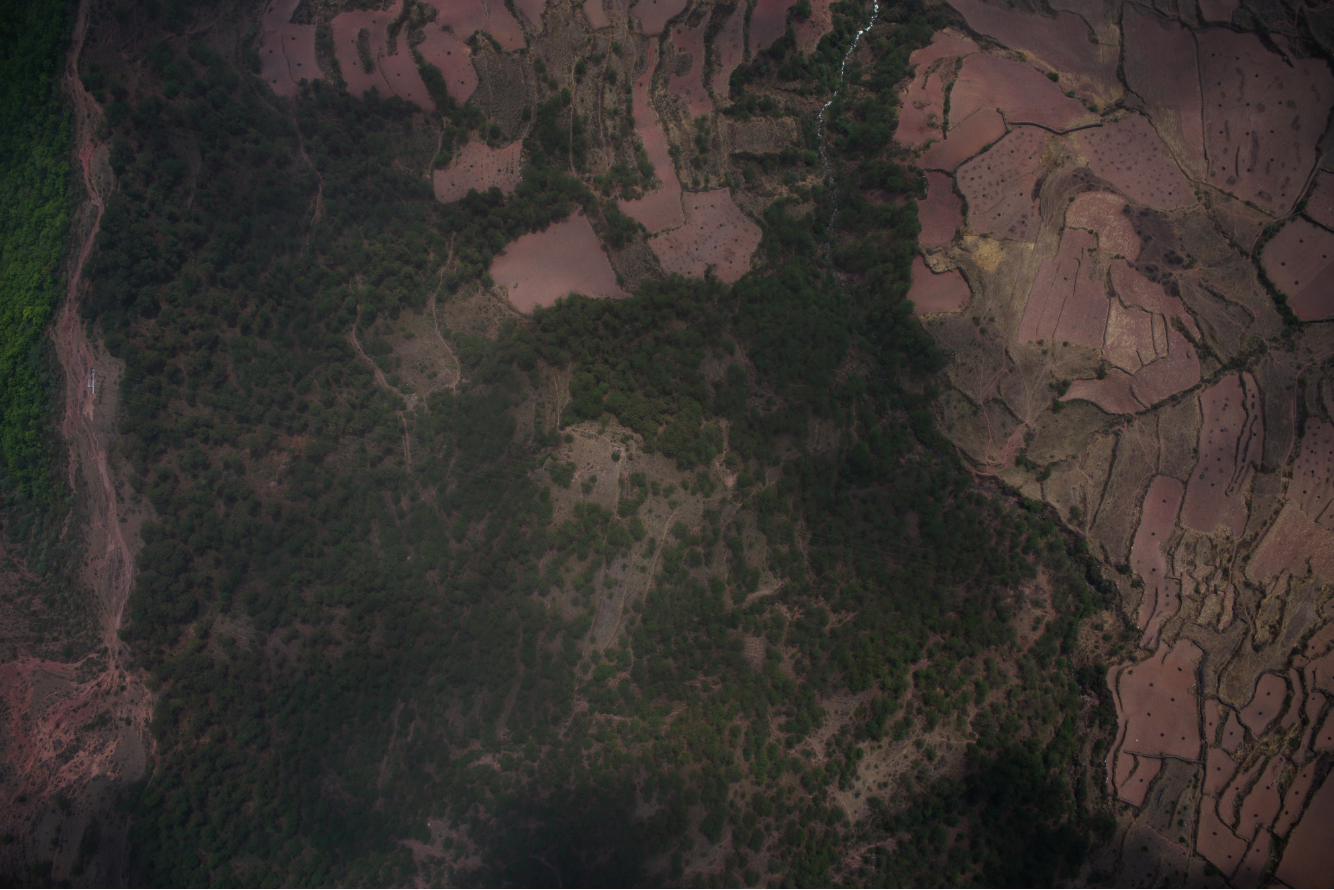展开全文• 题目：选择合适的基本灰度变换模型及其参数，通过该变换增强测试图像EXP2C.tif的全局对比度。...选择幂律（伽马）变换模型来增强图像的对比度，幂律变换的模型为   在实验中将用X来表示伽马值γ。 步骤： 1...
题目：选择合适的基本灰度变换模型及其参数，通过该变换增强测试图像EXP2C.tif的全局对比度。

说明：要求设置合适的模型参数，以保证对比度增强后的图像不会丢失过多的图像细节信息。

实现思路：

选择幂律（伽马）变换模型来增强图像的对比度，幂律变换的模型为

在实验中将用X来表示伽马值γ。

步骤：

1.将图像归一化为0~255的double的图像，以便计算；

2.将图像数据代入幂律变换公式C*(img .^ X)中，根据所给参数处理图像；

3.通过不断调整幂律变换的参数伽马值X，输出图像；

4.分别查看和对比不同参数输出的图像情况，找到一个最适合的伽马值；

5.分析处理结果：当伽马值X为3时，图像处理结果有点“偏淡”，即一些细节看不清；当伽马值X为4时，图像处理效果比较好，细节比较明显；当伽马值X为5时，图像处理结果中有些地方“太暗了”，效果没有伽马值X为4时好；

6.得出结论：通过比较各参数输出图像的对比度情况，得出参数伽马值X为4时处理效果最好。

实现效果：

在同一个图形界面上演示对比度线性拉伸前后的图像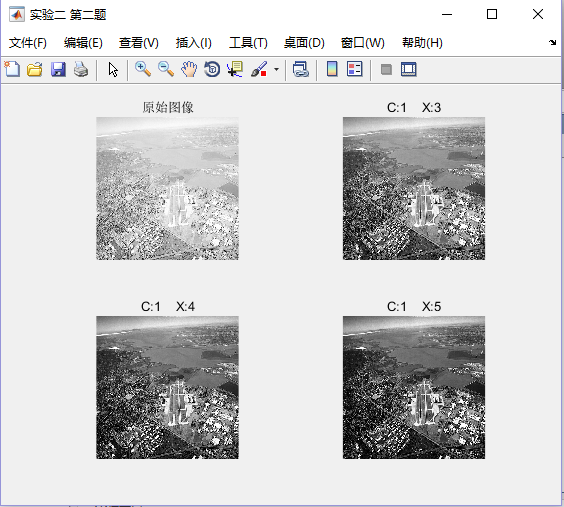图1 幂律变换各参数结果图

实现源代码：

function img2 = myImageMiLv(img1,C,X)

img = im2double(img1);
img2 = C*(img .^ X);%进行幂律变换
end


clc;
clear;

figure('NumberTitle', 'off', 'Name', '实验二 第二题');
subplot(2,2,1);
imshow(img1);
title('原始图像');

C = 1;
X = 3;
img2 = myImageMiLv(img1,C,X);
subplot(2,2,2);
imshow(img2);
title(['C:',num2str(C),'    X:',num2str(X)]);

C = 1;
X = 4;
img2 = myImageMiLv(img1,C,X);
subplot(2,2,3);
imshow(img2);
title(['C:',num2str(C),'    X:',num2str(X)]);

C = 1;
X = 5;
img2 = myImageMiLv(img1,C,X);
subplot(2,2,4);
imshow(img2);
title(['C:',num2str(C),'    X:',num2str(X)]);


展开全文matlab image
• 数字图像处理(Digital Image Processing)是通过计算机对图像进行...在数字图像处理学习笔记（八）中，已对图像增强之线性变换、对数变换、幂律变换、分段线性变换、灰度级分层等做过详细理论论述，本文将对上述理...图像处理 python pycharm 图像增强 学习笔记
• 幂律变换的基本形式为：，其中和是常数 有时考虑到偏移量，上式也写为。然而，偏移量是一般显示标定问题，因而作为一个结果，通常在上式中忽略不计。 与对数变换情况类似，部分值得幂律曲线将较窄范围的暗色值，...幂律变换 数字图像处理 Python
• 本文主要介绍对《数字图像处理》第三章书中示例图片实现 反转变换、对数变换以及伽马变换的代码 若要获取更多数字图像处理，python，深度学习，机器学习，计算机视觉等高清PDF以及 更多有意思的 分享，可搜一搜 微信...数字图像处理 图像增强 对数变换 幂律变换
• 对数变换： CImg&lt;int&gt; SrcImg; SrcImg.load_tiff("E:/Desktop/picture_process/Lenna/3/Fig0305(a)(DFT_no_log).tif"); SrcImg.display(); cimg_forXY(SrcImg, x, y) { SrcImg(x, y) = int...c++
• 幂律变换的基本形式为： （1） 其中c和为正常数。有时考虑到偏移量 可将式（1）写为。偏移量一般是显示标定问题，作为一个结果，通常在式（1）中忽略不计。对于不同的值，s与r的关系如图1所示。 图1 变换曲线 与...gamma校正 幂律变换
• 文章目录变换核磁共振图像代码实现遇到问题附代码： 变换 变换，点运算的一种，运算公式为s=crγ，其中，c和γ是正常数。 当γ<1，此时扩展低灰度级，压缩高灰度级，在正比函数上方，使图像变亮； 当...
• 灰度是指使用黑色调表示物体，用黑色为基准色，不同的饱和度的黑色来显示图像，灰度共有256级 灰度色，就是指纯白、纯黑以及两者中的一系列从黑到白的过渡色 如把有黑-灰-白连续变化的灰度值量化为256个灰度级...数字图像处理
• 彩色图像的增强处理在许多可携带设备中都是一个非常重要的过程，包括像平板、数字相机和智能手机等。但是，考虑到便携设备有限的存储容量和较低的计算能力，提高处理过程中的计算效率就成为了一个优先解决问题。这篇...图像识别
• 空间域处理包括灰度变换和空间滤波两类，作用是用来对图像进行增强...空间域指的是图像平面本身，这类图像处理技术直接操作图像中的像素。 空间域处理可以用下列表达式来表示：g(x,y) = T[ f(x,y) ]，其中f(x,y)是...
• 主要参考内容 ...图像增强：线性、 分段线性、 对数、 反对数、 幂律(伽马)变换、直方图均衡. 感谢各位博主分享，侵删 关于OpenCV和numpy模块 需要先导入cv2和numpy库，我的pycharm已经安装了anacond...python opencv
• 1、算法原理 伽马变换（幂律变换）是常用的灰度变换，是一种简单的图像增强算法。数学公式如下：（1） 式（1）中，r为输入的灰度值，取值范围为[0, 1]。C称为灰度缩放系数，用于整体拉伸图像灰度，通常取值为1。...幂律变换 图像增强
• ## 图像增强-伽马变换

千次阅读 2019-08-28 20:43:25
幂律变换主要用于图像的校正，对漂白的图片或者是过黑的图片进行修正，幂律变换的公式如下： 根据 γ的大小，主要可分为以下两种情况： γ > 1： 处理漂白的图片，进行灰度级压缩 γ < 1： 处理过黑的...opencv
• FPGA开源工作室FPGA/图像处理/创业/职场关注基于FPGA的灰度图像处理之幂律（伽马）变化1 背景知识幂律变换的基本形式为：----------------------------...
• 灰度变换图像中单个像素进行处理，它的数学表示： s = T(r). 其中s和r分别表示处理前后的像素值，T是把像素值r映射到s的一种变换。 一些基本的灰度变换函数：线性函数，对数函数，幂律函数。如下图： 反转变...图像处理 灰度变换 比特平面分层
• 目录背景知识灰度变换和空间滤波基础一些基本的灰度变换函数图像反转对数变换幂律（伽马）变换分段线性变换函数对比度拉伸灰度级分层比特平面分层直方图处理直方图均衡空间滤波基础空间滤波机理空间相关与卷积相关...数字图像处理
...